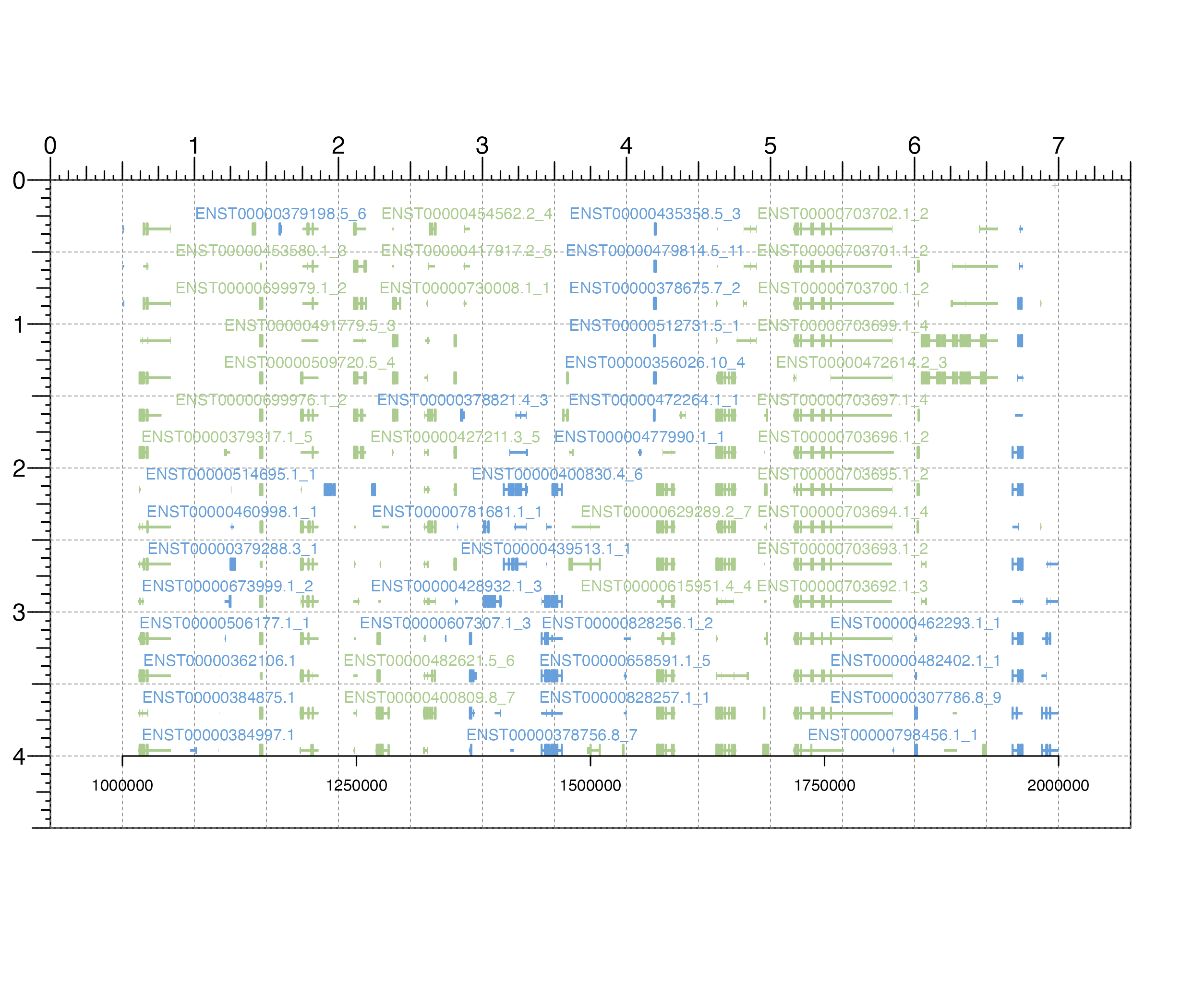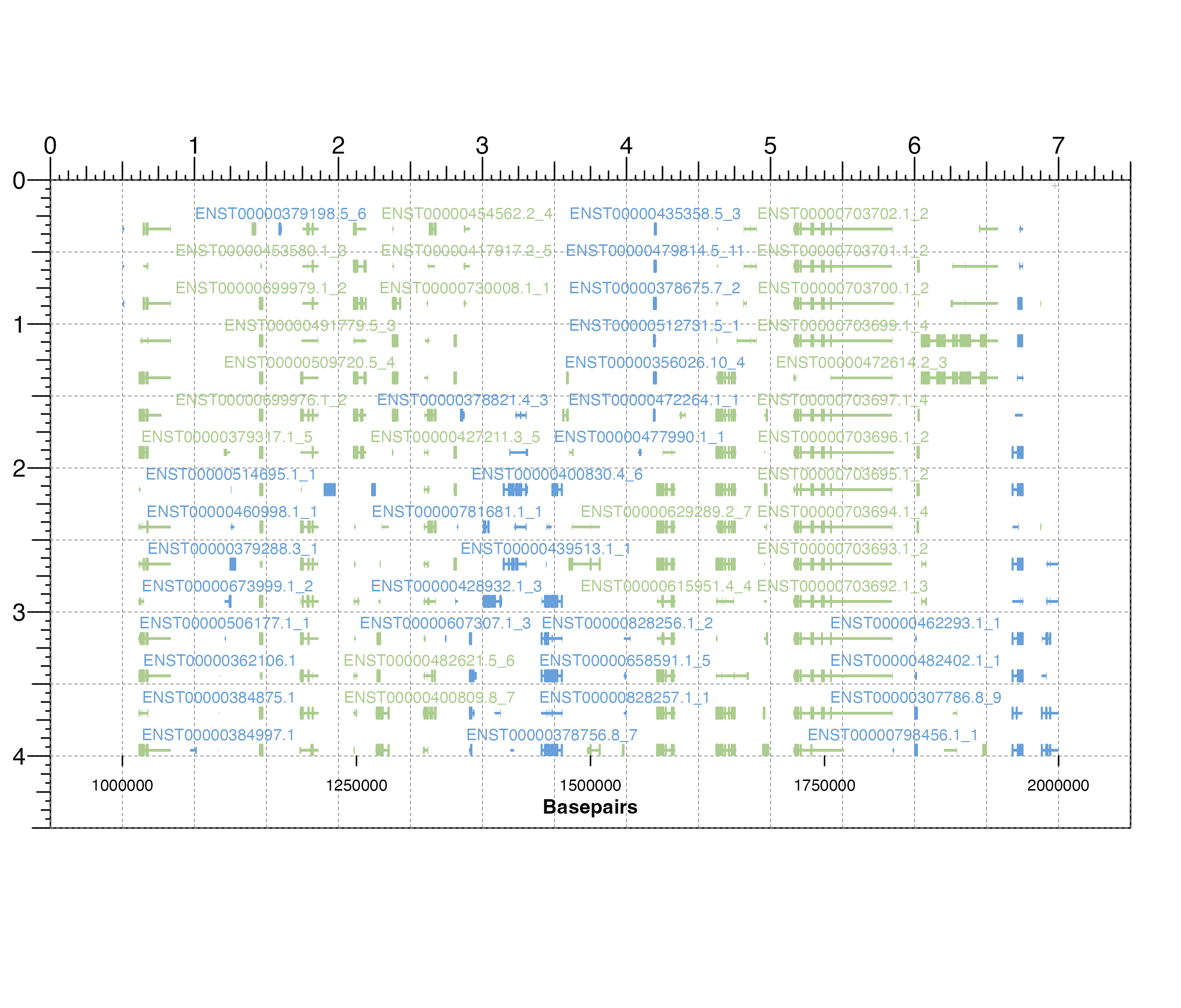Add an x-axis to a plot

annoXaxis(
plot,
at = NULL,
label = TRUE,
main = TRUE,
scipen = 999,
axisLine = FALSE,
params = NULL,
...
)

## Arguments

plot

Plot object to annotate with x-axis.

at

A numeric vector of x-value locations for tick marks.

label

A logical value indicating whether to draw the labels on the tick marks, or an expression or character vector which specify the labels to use. If not logical, must be the same length as the at argument.

main

A logical value indicating whether to draw the x-axis at the bottom of the plot. Default value is main = TRUE. Options are:

• TRUE: x-axis is drawn at the bottom of the plot.

• FALSE: x-axis is drawn at the top of the plot.

scipen

An integer indicating the penalty to be applied when deciding to print numeric values in fixed or exponential notation. Default value is scipen = 999.

axisLine

A logical value indicating whether to show the axis line. Default value is axisLine = FALSE.

params

An optional pgParams object containing relevant function parameters.

...

Additional grid graphical parameters. See gpar.

## Value

Returns a xaxis object containing relevant grob information.

## Examples

## Load transcript information
library("TxDb.Hsapiens.UCSC.hg19.knownGene")
library("org.Hs.eg.db")

## Create page
pageCreate(width = 7.5, height = 4.5, default.units = "inches")

## Plot gene transcripts
transcriptPlot <- plotTranscripts(
chrom = "chr1",
chromstart = 1000000,
chromend = 2000000,
assembly = "hg19",
x = 0.5, y = 0,
width = 6.5, height = 4,
just = c("left", "top"),
default.units = "inches"
)
#> Warning: Not enough plotting space for all provided elements. ('+' indicates elements not shown.)
#> transcripts[transcripts1]

## Add standard x-axis to transcript plot
annoXaxis(
plot = transcriptPlot,
at = c(1000000, 1250000, 1500000, 1750000, 2000000),
fontsize = 8
)#> xaxis[xaxis1]
plotText(
label = "Basepairs", fontsize = 10, fontface = "bold",
x = 3.75, y = 4.3, just = "top"
)
#> text[text1]## Hide page guides
pageGuideHide()